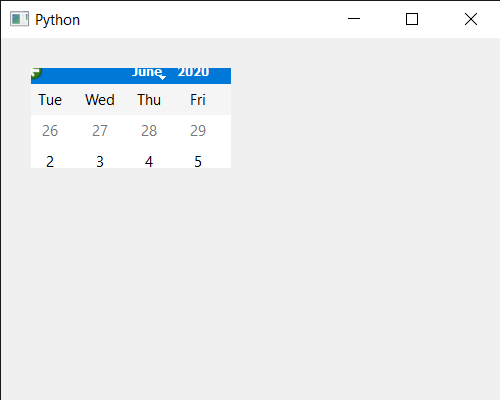# PyQt5 QCalendarWidget – Setting Mask

• Last Updated : 17 Jun, 2020

In this article we will see how we can set the mask to the QCalendarWidget. Mask are basically used to hide the calendar, with the help of mask user will not be able to see the whole calendar although the calendar will still exist but there will be mask on it.

It causes only the pixels of the calendar for which bitmap has a corresponding 1 bit to be visible. If the region includes pixels outside the rectangle of the calendar, window system controls in that area may or may not be visible, depending on the platform.

In order to do this we will use `setMask` method with the QCalendarWidget object.

Argument : It takes QRegion object or QBitMap object as argument

Return : It return None

Below is the implementation

 `# importing libraries``from` `PyQt5.QtWidgets ``import` `*` `from` `PyQt5 ``import` `QtCore, QtGui``from` `PyQt5.QtGui ``import` `*` `from` `PyQt5.QtCore ``import` `*` `import` `sys`` ` `# QCalendarWidget Class``class` `Calendar(QCalendarWidget):`` ` `    ``# constructor``    ``def` `__init__(``self``, parent ``=` `None``):``        ``super``(Calendar, ``self``).__init__(parent)``        ``self``.setMouseTracking(``True``)`` ` ` ` `class` `Window(QMainWindow):`` ` `    ``def` `__init__(``self``):``        ``super``().__init__()`` ` `        ``# setting title``        ``self``.setWindowTitle(``"Python "``)`` ` `        ``# setting geometry``        ``self``.setGeometry(``100``, ``100``, ``500``, ``400``)`` ` `        ``# calling method``        ``self``.UiComponents()`` ` `        ``# showing all the widgets``        ``self``.show()`` ` ` ` `    ``# method for components``    ``def` `UiComponents(``self``):`` ` `        ``# creating a QCalendarWidget object``        ``# as Calendar class inherits QCalendarWidget``        ``self``.calendar ``=` `Calendar(``self``)`` ` `        ``# setting cursor``        ``self``.calendar.setCursor(Qt.PointingHandCursor)`` ` `        ``# setting geometry to the calendar``        ``self``.calendar.setGeometry(``20``, ``20``, ``300``, ``240``)`` ` `        ``# QRect object``        ``rect ``=` `QRect(``10``, ``10``, ``200``, ``100``)`` ` `        ``# creating a QRegion``        ``region ``=` `QRegion(rect)`` ` `        ``# setting mask to the calendar``        ``self``.calendar.setMask(region)`` ` ` ` `# create pyqt5 app``App ``=` `QApplication(sys.argv)`` ` `# create the instance of our Window``window ``=` `Window()`` ` `# start the app``sys.exit(App.``exec``())`

Output :My Personal Notes arrow_drop_up